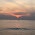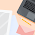Best answer of tcs codevita 2015 questions

Friends, you may have faced C++ questions in Codevita exam organised by TCS. Here is an answer of a question asked in tcs codevita. If you need more questions go to TCS codevita important questions 2015.

Friends, you may have faced C++ questions in Codevita exam organized by TCS. Here is an answer to a question asked in TCS codevita. If you need more questions go to TCS codevita important questions 2015.## TCS codevita Question

### Problem : Milk Man and His Bottles

A Milkman serves milk in packaged bottles of varied sizes. The possible size of the bottles are {1, 5, 7 and 10} litres. He wants to supply desired quantity using as less bottles as possible irrespective of the size. Your objective is to help him find the minimum number of bottles required to supply the given demand of milk.

#### Input Format:

• First line contains number of test cases N
• Next N lines, each contain a positive integer Li which corresponds to the demand of milk.

#### Output Format:

• For each input Li, print the minimum number of bottles required to fulfill the demand

#### Constraints:

• 1 <= N <= 1000
• Li> 0
• 1 <= i <= N

#### Sample Input and Output

 SNo. Input Output 1 2 17 65 2 7

Explanation:

Number of test cases is 2

In first test case, demand of milk is 17 litres which can be supplied using minimum of 2 bottles as follows
1 x 10 litres and
1 x 7 litres
In second test case, demand of milk is 65 litres which can be supplied using minimum of 7 bottles as follows
6 x 10 litres and
1 x 5 litres

Solution : Milk Man and His Bottles

#include <iostream>
using namespace std;
int minimum(int n1,int n2,int n3)
{
int a={n1,n2,n3},minim=a;
for(int i=0;i<3;i++)
{
if(minim>a[i])
minim=a[i];
}
return minim;
}
int f(int n,int no)
{
if(n==1||n==5||n==7||n==10)
return no+1;
if(n>1&&n<5)
return (f(n-1,no+1));
if(n>5&&n<7)
return (f(n-5,no+1));
if(n>7&&n<10)
return (f(n-7,no+1));
if(n>10)
{
return(minimum(f(n-7,no+1),f(n-10,no+1),f(n-5,no+1)));
}
cout<<"error";
return 0;
}
int main()
{int n,cases;
cout<<"Enter cases: ";
cin>>cases;
for(int i=0; i<cases; i++){
cout<<"Enter demand: ";
cin>>n;
cout<<"minimum no of bottles required are: ";
cout<<f(n,0)<<"\n";
}
return 0;
}

Mr. Sai kalyan gave me the code for this milkman question in java. Here it is

/* package codechef; // don't place package name! */
import java.util.*;
import java.lang.*;
import java.io.*;
/* Name of the class has to be "Main" only if the class is public. */
class Milkman
{
public static void main (String[] args) throws java.lang.Exception
{
int[] bottles = {10,7,5,1};
int[] result = new int[test_case];
int milk,lts,btl,j;
for(int i=0; i<test_case;i++)
{
j=0;
btl=0;
while(milk>0)
{
lts   = milk/bottles[j];
milk -= bottles[j]*lts;
j++;
btl += lts;
}
result[i] = btl;
}
for(int i=0;i<test_case;i++)
System.out.println(result[i]);
}
}

The Codevita problem code is well understood if you have any problem in any of the codes give your suggestion in the comment section. I will be Happy to Help you.

Help others by Sharing this post. Thank you.BLOGGER: 26
1.Hope you will get some help for mor info go to tcs portal campus commune

2.Here is my solution in java
import java.util.*;
class Milk
{
public static void main(String[] args)
{
Scanner sc=new Scanner(System.in);
int n,t,h=0,s;
s=sc.nextInt();
if(s<1||s>1000){System.exit(0);}
int a[]=new int[s];
for(int i=0;i<s;i++)
{
n=sc.nextInt();
if(n<0){System.exit(0);}
if(n<10)
{
h=0;
t=n;
}
else
{
h=n/10;
t=n%10;
}
if(t==1||t==5||t==7)
a[i]=h+1;
else if(t==2||t==6||t==8)
a[i]=h+2;
else if(t==3||t==9)
a[i]=h+3;
else if(t==4)
a[i]=h+4;
else
a[i]=h;
}
for(int i=0;i<s;i++)
System.out.println(a[i]);
}
}

3.Great loved your Code in Java is is working well.

4.The solution provided here is not correct. Take the case of 14. This program outputs 5. But the correct answer should be 2(two 7 L bottles)...

5.If you still unsatisfied please tell your doubt. I will see to it.

6.solution is wrong !!!!
suppose demand is of 19L...
so as per these code no. of bottles required is 4..
but instead it can be reduced to just 2 bottles if we use two 10 L bottles.

1.you were wrong..

19L needs 4 only...

but it is wrong for
22 32 42 52 etc

7.if we can leave any bottle a little empty , then the code becomes easy and its not much logical program.

btw

case 1 (every bottles are not full) :

import java.util.Scanner;

public class milk2
{
public static void main(String args[])
{
int vector[] = new int,i,nob;
Scanner obj = new Scanner(System.in);

int N = obj.nextInt();

if(N<=1000)
{
for(i=0;i<N;i++)
{
vector[i] = obj.nextInt();
}
System.out.println();
for(i=0;i<N;i++)
{
if(vector[i]%10 == 0)
System.out.println(vector[i]/10);
else
System.out.println((vector[i]/10)+1);
}
}
}
}

case 2 (every bottles are full) :

import java.util.Scanner;

public class milkman
{
public static void main(String args[])
{
int vector[] = new int,i,nob;
Scanner obj = new Scanner(System.in);

int N = obj.nextInt();

if(N<=1000)
{
for(i=0;i<N;i++)
{
vector[i] = obj.nextInt();
}
System.out.println();
for(i=0;i<N;i++)
{
if(vector[i]<5)
nob = vector[i];
else if(vector[i]==9)
nob = 3;
else if((vector[i]%10)==0)
nob = vector[i]/10;
else if((vector[i]-1)%10==0 || (vector[i]-2)%10==0 || (vector[i]-4)%10==0 || (vector[i]-5)%10==0 || (vector[i]-7)%10==0 )
nob = (vector[i]/10) + 1;
else
nob = (vector[i]/10) + 2;
System.out.println(nob);
}
}
}
}

:-)

8.How about using two 7 liter bottles? You want minimum number of bottles to use, right? Why are you using 5 bottles?

9.This is not a correct solution! It gives wrong answers for 14 , 24 and many more.

10.it can also be done with the help of dp for efficient solution

11.Yes, my friend you were right. Now I have changed that code, & updated above

12.I have updated the post with the new code. Hope this will help you. Thank you

13.#include

void main(){
int tests,count=0;
int i,value,n;
scanf("%d",&tests);
for(i=0;i<tests;i++){
scanf("%d",&value);
n=value;
if(n/10 != 0){
count += n/10;
value = value%10;
}
n=value;
if(n/7 != 0){
count += n/7;
value = value%7;
}
n=value;
if(n/5 != 0){
count += n/5;
value = value%5;
}
count +=value;
printf("%dn",count);
count=0;
}

}

1.Nice one dude

2.Nice one. But not works for all cases that is the problem in the above code

14.The solution to this problem is logical, not dp or greedy algorithms or any other sort of algorithm. If you think about it, you can easily deduce that in every case, the minimum number of bottles needed is = [(litres of milk)/(quantity of largest bottle)+1].
So, in this case, it is = [((litres of milk)/10)+1].
Problem solved in one line of code.

1.2.Code fails for 10,20,30.....etc

3.your logic [((litres of milk)/10)+1] is correct when we have chance to left one bottle partial empty.So your answer may fail for some cases like 19L,28L etc

15.codevista milkman

/*My solution in c++ assuming bottles cannot be incompletely filled:*/
#include
using namespace std;

int minimum(int n1,int n2,int n3)
{
int a={n1,n2,n3},minim=a;
for(int i=0;i<3;i++)
{
if(minim>a[i])
minim=a[i];
}
return minim;
}

int f(int n,int no)
{

if(n==1||n==5||n==7||n==10)
return no+1;

if(n>1&&n<5)
return (f(n-1,no+1));

if(n>5&&n<7)
return (f(n-5,no+1));

if(n>7&&n<10)
return (f(n-7,no+1));

if(n>10)
{
return(minimum(f(n-7,no+1),f(n-10,no+1),f(n-5,no+1)));
}

cout<<"error";
return 0;
}

int main()
{int n;
cout<<"enter demand";
cin>>n;
cout<<"minimum no of bottles are";
cout<<f(n,0);

return 0;
}
/*so o/p for 32 will be 4 not 5 which is according to the above code
As 10*2+7*1+5*1=32
so 4.
This code does not have good time complexity but it will never fail.
*/

16.class MilkMan
{
public static void main(String[] args)
{
int n;
//System.out.print("Enter the number of cases : ");
java.util.Scanner sc= new java.util.Scanner(System.in);
n=sc.nextInt();
while(n>0&&n<1000)
{
int Li=0,ten=0,seven=0,five=0,one=0,total=0,zero,four=0,fourteen=0,twelve=0,nineteen=0,thirteen=0;
//System.out.print("Enter the quantity of milk : ");
Li=sc.nextInt();
if(Li==4)
{
four=4;
Li=Li-4;
}
if(Li%10==4)
{
fourteen=2;
Li=Li-fourteen*7;
}
if(Li>=10)
{
if((Li-12)%10==0)
{

twelve=2;
Li=Li-12;
ten=Li/10;

Li=Li-ten*10;
}
else if((Li-13)%10==0)
{

thirteen=3;
Li=Li-13;
ten=Li/10;

Li=Li-ten*10;
}
else if((Li-19)%10==0)
{

nineteen=3;
Li=Li-19;
ten=Li/10;

Li=Li-ten*10;
}

else if((Li-14)%10==0)
{

fourteen=2;
Li=Li-14;
ten=Li/10;

Li=Li-ten*10;
}
else
{
ten=Li/10;
Li=Li-ten*10;
}
}
if(Li>=7)
{
seven=Li/7;
Li=Li-seven*7;
}
if(Li>=5)
{
five=Li/5;
Li=Li-five*5;
}
if(Li>=1)
{
one=Li;
}
total=ten+seven+five+one+fourteen+four+twelve+nineteen+thirteen;
//System.out.print("Number of bottles required : "+total+"\n");
System.out.println(total);
n--;
}

}
}

17.18.//Solution in C
#include
int main()
{
int N, size[]={1,5,7,10}, in, out={0}, i=0, j=3;
printf("No of test cases:\n");
scanf("%d", &N);
printf("Inputs:\n");
for(i=0;i0 && j>=0)
{
if(in[i]>=size[j])
{
in[i]-=size[j];
out[i]++;
}
else
j--;
}
}
printf("Outputs:\n");
for(i=0;i<N;i++)
{
printf("%d\n", out[i]);
}
return 0;
}

19.This is the c version of the solution of TCS codevita milkman question

#include studio.h

int minimum(int n1,int n2,int n3)
{
int a={n1,n2,n3},minim=a;
for(int i=0;i<3;i++)
{
if(minim>a[i])
minim=a[i];
}
return minim;
}
int f(int n,int no)
{
if(n==1||n==5||n==7||n==10)
return no+1;
if(n>1&&n<5)
return (f(n-1,no+1));
if(n>5&&n<7)
return (f(n-5,no+1));
if(n>7&&n<10)
return (f(n-7,no+1));
if(n>10)
{
return(minimum(f(n-7,no+1),f(n-10,no+1),f(n-5,no+1)));
}
printf("error");
return 0;
}
int main()
{int n,cases;
printf("Enter cases: ");
scanf("%d", &cases);
for(int i=0; i<cases; i++){
printf("Enter demand: ");
scanf("%d", &n);
printf("minimum no of bottles required are: ");

printf("%d\n",f(n,0));
}
return 0;
}

20.The code should be:
#include
#include
int main ()
{
int a ={1,5,7,10};
int l,n;
printf ("No of cases\n");
scanf ("%d",&n);
while (n>0)
{
int c=0;
printf ("Enter demand\n");
scanf ("%d",&l);
for (i=3;i>=0;i--)
{
c=c+(l/a [i]);
l=l%a [i];
}
n--;
printf ("Total bottles=%d",c);
}
}
Sample output:
No of cases
3
Enter demand 19
Total bottles=4
Enter demand 32
Total bottles=5
Enter demand 75
Total bottles=8

## Related Articles

Name

ltr
item
MindxMaster: Best answer of tcs codevita 2015 questions
Best answer of tcs codevita 2015 questions
Friends, you may have faced C++ questions in Codevita exam organised by TCS. Here is an answer of a question asked in tcs codevita. If you need more questions go to TCS codevita important questions 2015.
https://1.bp.blogspot.com/-ZtKwGBt_Lww/VcWjBEJOALI/AAAAAAAAAV0/wpsSEdV6CEI/s1600/2.-Code-Vita-2015.jpg
https://1.bp.blogspot.com/-ZtKwGBt_Lww/VcWjBEJOALI/AAAAAAAAAV0/wpsSEdV6CEI/s72-c/2.-Code-Vita-2015.jpg
MindxMaster